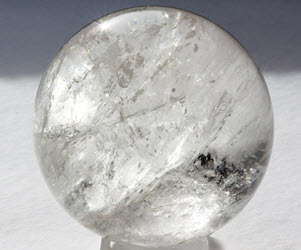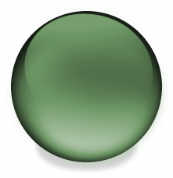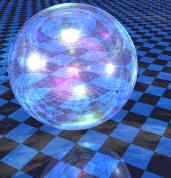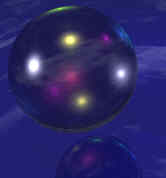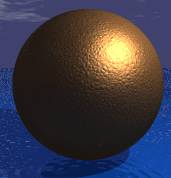# Spheres Overview

Author:
Christine
Topic:
Sphere
Sphere FactsNotice these interesting things:
• It is perfectly symmetrical
• All points on the surface are the  same distance "r" from the center
• It has no edges or  vertices (corners)
• It has one surface  (not a "face" as it isn't flat)Glass Sphere.  Balls and marbles are shaped like spheres. Largest Volume for Smallest Surface: Of all the shapes, a sphere has the smallest surface area for a volume. Or put another way it can contain the greatest volume for a fixed surface area. Example: if you blow up a balloon it naturally forms a sphere because it is trying to hold as much air as possible with as small a surface as possible   In NatureThe sphere appears in nature when a surface wants to be as small as possible. Examples include bubbles and water drops. Can you think of more?
 The Earth: The Planet Earth, our home, is nearly a sphere, except that it is squashed a little at the poles.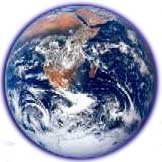It is a spheroid, which means it just misses out on being a sphere because it isn't perfect in one direction (in the Earth's case: North-South)
Volume and Surface AreaSurface Area = 4 × π × r2 Example: r = 5 Surface Area= 4 × π × r2   ﻿ ﻿ = 4 × π × 52 ﻿ ﻿  = 4 × π × 25   ﻿ ﻿ = 100 π   ﻿ ﻿ ≈ 314   Volume = (4/3) × π × r3 Example: r = 5 Volume= (4/3) × π × r3   ﻿ = (4/3) × π × 53   ﻿ = (4/3) × π × 125   ﻿ ≈ 524 Hemisphere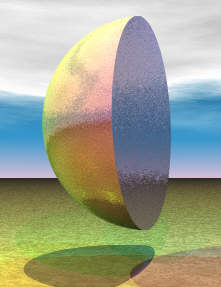A hemisphere is an exact half of a sphere. Other Cool Spheres